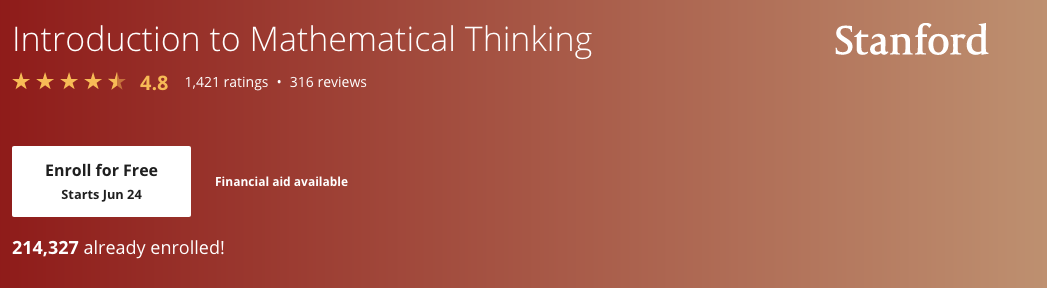Introduction to mathematics

Mathematical language
• Object a has property P.
• Every object of type T has property P.
• There is an object of type T having property P.
• If statement A, then statement B.
Logical combinators

AND

• ^ - wedge.
• Conjunction.
• Example: X ^ Y
• X and Y are called conjuncts.

OR

• V - disjunction
• X V Y

NOT

• Negation: ~ or ¬
• Example: ¬(X)